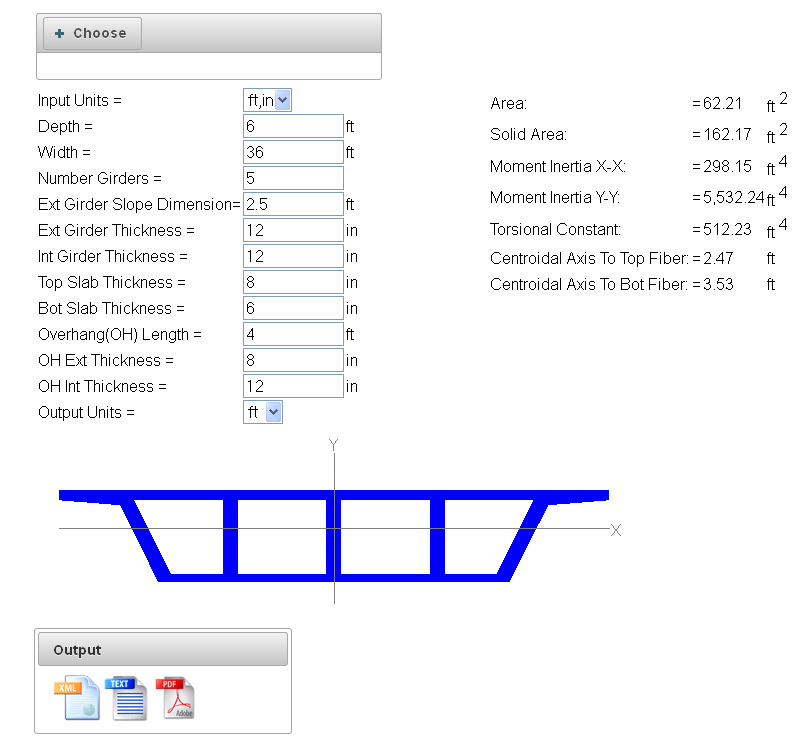# Area Section Properties By X, Y Coordinates

The properties of a polygon can be calculated using the equations below. The shape can be solid or have one or more “holes.” The coordinates for the solid section are input clockwise and holes or voids are entered anti-clockwise.

Input

Equations$A=-\sum_{i=0}^{n-1}{\frac{(y_{i+1}-y_i)(x_{i+1}+x_i)}{2} }$$\bar{x}=\frac{-1}{A}\sum_{i=0}^{n-1} ( \frac{y_{i+1}-y_i}{8})[ (x_{i+1}+x_i)^2+\frac{(x_{i+1}-x_i)^2}{3}]$$\bar{y}=\frac{1}{A}\sum_{i=0}^{n-1} ( \frac{x_{i+1}-x_i}{8})[ (y_{i+1}+y_i)^2+\frac{(y_{i+1}-y_i)^2}{3}]$$I_x=\sum_{i=0}^{n-1} [\frac{{(x_{i+1}-x_i)}{(y_{i+1}+y_i)}}{24}][{(y_{i+1}+y_i)^2}+{(y_{i+1}-y_i)^2}]$$I_y=-\sum_{i=0}^{n-1} [\frac{{(y_{i+1}-y_i)}{(x_{i+1}+x_i)}}{24}][{(x_{i+1}+x_i)^2}+{(x_{i+1}-x_i)^2}]$$I_{\bar{x}}=I_x-A\bar{y}^2$$I_{\bar{y}}=I_y-A\bar{x}^2$

Definitions$A$ – is the area of the shape$\bar{x}$ – is the x coordinate of the centroid$\bar{y}$ – is the y coordinate of the centroid$I_x$ – is the moment of inertia about the x axis$I_y$ – is the moment of inertia about the y axis$I_{\bar{x}}$ – is the moment of inertia about the x axis translated to the centroid$I_{\bar{y}}$ – is the moment of inertia about the y axis translated to the centroid

Web Application

Bridge Automation’s Section Property Calculator uses the above equations and can be found at this link.